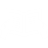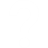# Income statement

The Income Statement (also called the profit and loss statement or profit and loss account) focuses on a company’s revenue and expenses for a particular period of time (e.g. monthly, quarterly, or annually).

An income statement is based on the following equation (Profit = Income – Expenses).## Quiz

Try the following questions to check what you have understood.

• A company has annual Sales of \$328,000 with Cost of Goods Sold \$142,000. What is the company’s Gross Profit?
• \$470,000
• Try again. Don’t forget, (Annual sales) – (Cost of Goods) = (Gross Profit).
• \$186,000
• Yes, that’s right. (Annual sales) – (Cost of Goods) = (Gross Profit).
• The same company (over the same period of time) spends \$76,000 on Salaries and \$11,000 on Utilities. What is their income before tax?
• \$99,000
• That’s correct.(Gross Profit) – (Salaries) – (Utilities) = (Income before tax).
• \$87,000
• Try again. (Gross Profit) – (Salaries) – (Utilities) = (Income before tax).
• \$65,000
• Try again. (Gross Profit) – (Salaries) – (Utilities) = (Income before tax).
• If income tax is 20%, what is the same company’s net income?
• \$79,200
• Well done! (Income before tax) – (Income before tax x 20%) = Net Income.
• \$72,900
• Try again (Income before tax) – (Income before tax x 20%) = Net Income.
• Your company has annual Sales of \$447,000 and Cost of Goods Sold is \$199,000. What is your Gross Profit?
• \$646,000
• Try again. Remember, (Annual sales) – (Cost of Goods) = (Gross Profit).
• \$248,000
• Yes, that’s correct. (Annual sales) – (Cost of Goods) = (Gross Profit).
• For the same company (over the same period of time), Salaries are \$104,000 and Utilities cost \$18,000. What is your Income before tax?
• \$122,000
• Try again. (Gross Profit) – (Salaries) – (Utilities) = (Income before tax).
• \$126,000
• That’s right. (Gross Profit) – (Salaries) – (Utilities) = (Income before tax).
• \$370,000
• Try again. (Gross Profit) – (Salaries) – (Utilities) = (Income before tax).
• Now, if income tax is 20%, what is your Net Income
• \$25,200
• Incorrect. This is how much Income Tax you have to pay i.e. (Income before tax x 20%).
• \$100,800
• Well done!. (Income before tax) – (Income before tax x 20%) = Net Income.## Income statement activity

• Draw up an income statement based on the following figures to determine net profit:
 Sales 629,000 Cost of goods sold 293,000 Salary expense 127,000 Utilities expense 24,000 Income tax 20%

Item Income Expenditure
Sales
Less Cost of Goods Sold
Gross Profit
Less Salary expense
Less Utilities expense
Income before tax
Less Income Tax Expense
Net Profit